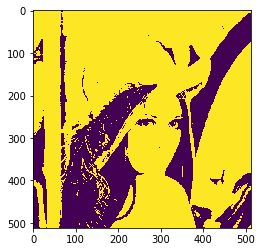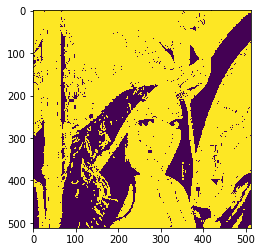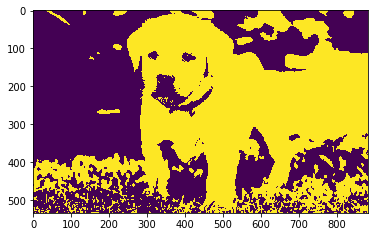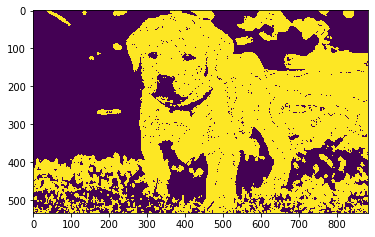# Mahotas – Conditional Dilating Image

• Last Updated : 30 Jul, 2021

In this article we will see how we can do conditional dilating of the image in mahotas. Dilation adds pixels to the boundaries of objects in an image, while erosion removes pixels on object boundaries. The number of pixels added or removed from the objects in an image depends on the size and shape of the structuring element used to process the image. We use mahotas.morph.dilate method to do normal dilating.

In this tutorial we will use “lena” image, below is the command to load it.

`mahotas.demos.load('lena')`

Below is the lena imageIn order to do this we will use mahotas.cdilate method
Syntax : mahotas.cdilate(img, c_grey, Bc={3×3 cross}, n=1)
Argument : It takes image object, conditional image as compulsory argument, element structure and iteration number are optional argument
Return : It returns image object

Note : Input image should be filtered or should be loaded as grey

In order to filter the image we will take the image object which is numpy.ndarray and filter it with the help of indexing, below is the command to do this

`image = image[:, :, 0]`

Below is the implementation

## Python3

 `# importing required libraries``# importing required libraries``import` `mahotas``import` `mahotas.demos``from` `pylab ``import` `gray, imshow, show``import` `numpy as np`` ` `# loading image``img ``=` `mahotas.demos.load(``'lena'``)` `# grey image``g ``=` `img[:, :, ``1``]` `# multiplying grey image values``g ``=` `g ``*` `100`` ` `# filtering image``img ``=` `img.``max``(``2``)`` ` `# otsu method``T_otsu ``=` `mahotas.otsu(img)``  ` ` ` `# image values should be greater than otsu value``img ``=` `img > T_otsu`` ` `print``(``"Image threshold using Otsu Method"``)`` ` `# showing image``imshow(img)``show()`` ` `# dilating image using conditional grey image``dilate_img ``=` `mahotas.cdilate(img, g)`` ` `# showing dilated image``print``(``"Dilated Image"``)``imshow(dilate_img)``show()`

Output :

`Image threshold using Otsu Method``Dilated Image`Another example

## Python3

 `# importing required libraries``import` `mahotas``import` `numpy as np``from` `pylab ``import` `gray, imshow, show``import` `os`` ` `# loading image``img ``=` `mahotas.imread(``'dog_image.png'``)``    ` `# grey image``g ``=` `img[:, :, ``2``]` `# multiplying grey image values``g ``=` `g ``*` `100`` ` `# filtering image``img ``=` `img[:, :, ``0``]`` ` `# otsu method``T_otsu ``=` `mahotas.otsu(img)``  `  `# image values should be greater than otsu value``img ``=` `img > T_otsu`` ` `print``(``"Image threshold using Otsu Method"``)`` ` `# showing image``imshow(img)``show()`` ` `# dilating image using conditional grey image``dilate_img ``=` `mahotas.cdilate(img, g)`` ` `# showing dilated image``print``(``"Dilated Image"``)``imshow(dilate_img)``show()`

Output :

`Image threshold using Otsu Method ``Dilated Image`My Personal Notes arrow_drop_up